# 耍杂技的牛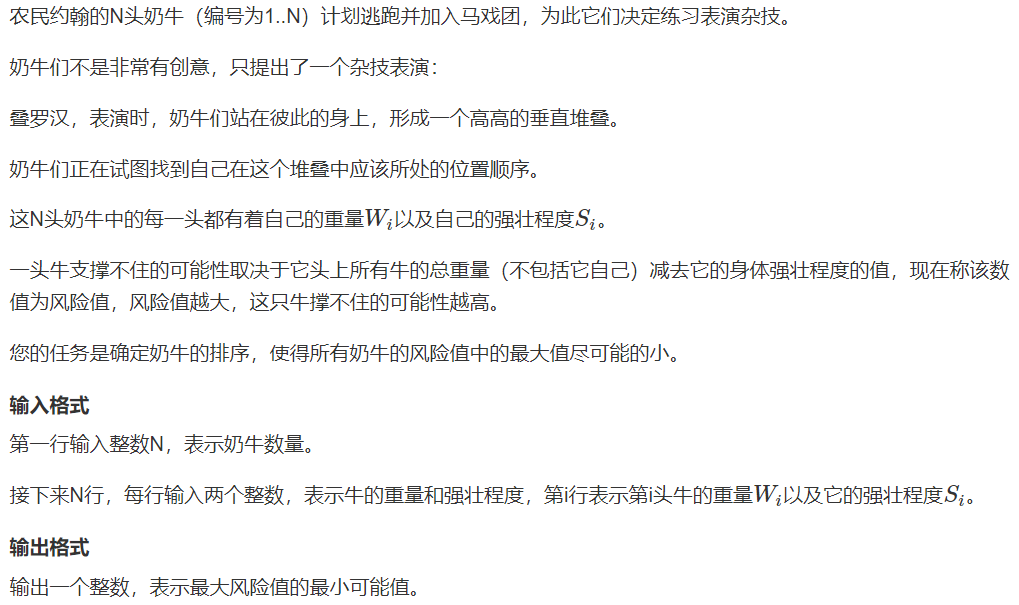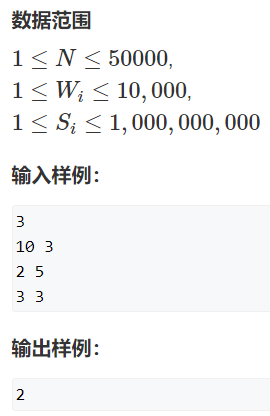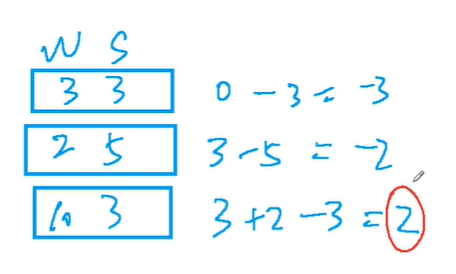题目意思就是，我们要安排一个从上到下的顺序，使得牛按照这个顺序叠高高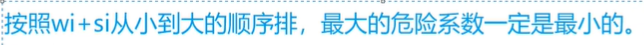证明：

然后如果我们交换这两头牛的话，看看会有什么影响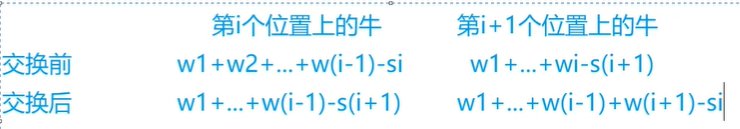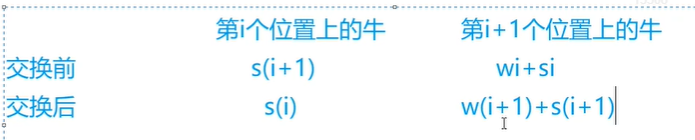因此si 和 w(i + 1) + s(i + 1)中的最大值一定小于 wi + si

 1 #include <bits/stdc++.h>
2 using namespace std;
3 typedef pair<int, int> PII;
4 const int N = 50010;
5 PII cow[N];
6 int main() {
7     int n;
8     cin >> n;
9     for (int i = 0; i < n; i++) {
10         int w, s;
11         cin >> w >> s;
12         cow[i] = {w + s, s};
13     }
14     sort(cow, cow + n);
15     int res = -2e9, sum = 0;
16     //先把最小值取为-INF
17     //sum是每头牛上面的牛重量之和
18     for (int i = 0; i < n; i++) {
19         int s = cow[i].second, w = cow[i].first - s;
20         res = max(res, sum - s);
21         sum += w;
22     }
23     cout << res << endl;
24     return 0;
25 }

posted @ 2020-11-24 20:09  kyk333  阅读(117)  评论(0编辑  收藏  举报